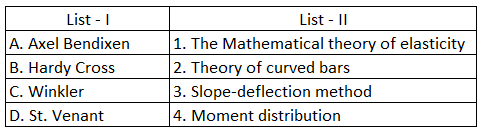# ISRO Scientist or Engineer Civil 2013

Instructions

For the following questions answer them individually

Question 1

# A cohesive soil yields a maximum dry density of $$18kN/m^3$$ during a standard proctor compaction test. If the specific gravity is 2.65, what would be its void ratio? (Adopt unit of water as $$10kKN/m^3$$)Question 2

# A footing $$2m \times 1m$$ exerts a uniform pressure of $$150kN/m^2$$ on the soil. Assuming a load dispersion of 2 vertical to 1 horizontal, the average vertical stress in $$(kN/m^2)$$ at 1.0m below the footing isQuestion 3

# Match List-I with List-ll and select the correct answer using the code given below the lists :Question 4

# The standard size of brick isQuestion 5

# The velocity of flow of water in a pipe of 150 mm dia is 0.3m/sec, a diaphragm with a central hole 80mm in diameter is placed in the pipe obstructing the flow. With coefficient of contraction $$C_c = 0.60, the loss of head from Vena Contracta to a point downstream will beQuestion 6 # If the depletion of oxygen is found to be 5 ppm after incubating a 2.5% solution of sewage sample for 5 days at$$20^\circ C$$, B.O.D of the sewage isQuestion 7 # A trapezoidal section of an open channel has side slope 2H:1V. If bottom width is 'b’ and depth ‘d’, the relation between b & d for most economical trapezoidal section of the channel is:Question 8 # If the difference in elevation of an edge of the pavement 9m wide and its crown is 15 cm, the camber of the pavement isQuestion 9 # The scour depth D of a river during flood, may be calculated from the Lacey’s equationQuestion 10 # The Glycerine is flowing at$$25^\circ C in a pipe of diameter 150mm with a velocity of 3.6m/s. The flow isOR